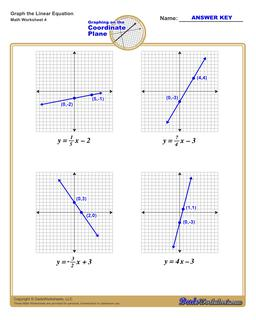PLEASE GO BACK AND USE THE BIG BLUE 'PRINT' BUTTON ON THE PAGE TO PRINT THE WORKSHEET CORRECTLY!Sorry for the trouble! The browser won't print the embedded worksheet PDF directly using the normal 'Print' command in the file menu, so you need to click the big 'Print' button to send just the worksheet and not the surrounding page to the printer.

Math Worksheets: Linear Equations: Linear Equations: Graphing Linear Equations (Fourth Worksheet)Graphing Linear Equations (Fourth Worksheet)

PropertyValue
DescriptionGraphing Linear Equations: Each graphing linear equations worksheet on this page has four coordinate planes and equations in slope-intercept form, and includes an answer key showing the correct graph. (Fourth Worksheet)
Resource TypeWorksheet